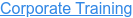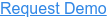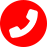# Data Science : Interview questions & answers

by Pritha Radhakrishnan, on May 31, 2023 4:24:42 PM

## 1. What is data science, and why is it important?

Ans: Data science is an interdisciplinary field that uses scientific methods, processes, algorithms, and systems to extract knowledge and insights from structured and unstructured data. It is important because it enables businesses to make data-driven decisions, uncover patterns and trends, and derive actionable insights to drive innovation and optimize processes.

## 2. What are the key steps involved in a data science project?

Ans: The key steps in a data science project include understanding the problem, acquiring and preprocessing data, exploratory data analysis, feature engineering, model selection and training, model evaluation, and deployment.

## 3. What is the difference between supervised and unsupervised learning?

Ans: In supervised learning, the algorithm learns from labeled training data to make predictions or classifications. In unsupervised learning, the algorithm learns patterns and relationships from unlabeled data without specific guidance or predefined outcomes.

## 4. What evaluation metrics would you use  to assess a regression model?

Ans: Common evaluation metrics for regression models include mean squared error (MSE), root mean squared error (RMSE), mean absolute error (MAE), and R-squared.

## 5. How do you handle missing values in a dataset?

Ans: Missing values can be handled by either removing rows with missing values, imputing them with mean or median values, or using advanced techniques like regression imputation or multiple imputation.

## 6. What is the curse of dimensionality, and how does it affect machine learning models?

Ans: The curse of dimensionality refers to the challenges and limitations that arise when working with high-dimensional data. It can lead to increased computational complexity, overfitting, and decreased model performance if not properly addressed through dimensionality reduction techniques.

## 7. Explain the bias-variance trade-off.

Ans: The bias-variance trade-off is a fundamental concept in machine learning. Bias refers to the error introduced by approximating a real-world problem with a simplified model, while variance refers to the model's sensitivity to variations in the training data. It is necessary to strike a balance between bias and variance to achieve optimal model performance.

## 8. What are the assumptions of linear regression?

Ans: Linear regression assumes a linear relationship between the dependent variable and independent variables, independence of observations, homoscedasticity (constant variance of errors), and absence of multicollinearity (no high correlation between independent variables).

## 9. What is feature selection, and why is it important?

Ans: Feature selection is the process of identifying the most relevant features or variables that contribute significantly to the predictive power of a model. It is important to remove irrelevant or redundant features, improve model interpretability, reduce overfitting, and enhance computational efficiency.

## 10. What are the different resampling methods in machine learning?

Ans: Resampling methods include cross-validation, bootstrapping, and hold-out validation. Cross-validation is commonly used to estimate model performance by partitioning the data into training and validation sets. Bootstrapping involves sampling with replacement to create multiple datasets for model training and evaluation. Hold-out validation splits the data into training and testing sets for performance evaluation.

## 11. What is regularization, and why is it used in machine learning?

Ans: Regularization is a technique used to prevent overfitting in machine learning models. It introduces a penalty term to the loss function, which controls the complexity of the model by shrinking the coefficients towards zero. Regularization techniques include L1 regularization (Lasso) and L2 regularization (Ridge).

## 12. How do you handle imbalanced datasets in classification problems?

Ans: Imbalanced datasets can be handled by techniques such as oversampling the minority class, undersampling the majority class, using ensemble methods like random forest or gradient boosting, or using specialized algorithms like SMOTE (Synthetic Minority Over-sampling Technique).

## 13. Explain the difference between bagging and boosting.

Ans: Bagging (bootstrap aggregating) is an ensemble learning technique where multiple models are trained independently on different bootstrap samples of the training data, and their predictions are averaged. Boosting is an ensemble learning technique where models are trained sequentially, with each subsequent model focusing on the errors of the previous model to improve overall prediction accuracy.

## 14. What are the different types of clustering algorithms?

Ans: The different types of clustering algorithms include k-means clustering, hierarchical clustering, DBSCAN (Density-Based Spatial Clustering of Applications with Noise), and Gaussian mixture models.

## 15. How does the Naive Bayes algorithm work?

Ans: The Naive Bayes algorithm is a probabilistic classifier based on Bayes' theorem. It assumes that the features are conditionally independent given the class label. It calculates the posterior probability of each class based on the prior probabilities and the likelihood of the features.

## 16. What is the purpose of A/B testing in data science?

Ans: A/B testing is a statistical hypothesis testing technique used to compare two versions (A and B) of a webpage, feature, or product to determine which performs better. It helps in making data-driven decisions by evaluating the impact of changes and identifying the optimal version based on user behavior or desired metrics.

## 17. How do you handle outliers in a dataset?

Ans: Outliers can be handled by either removing them, transforming the data using techniques like logarithmic or winsorization, or using robust statistical models that are less sensitive to outliers.

## 18. What is cross-validation, and why is it important?

Ans: Cross-validation is a technique used to evaluate the performance of a model by partitioning the data into training and validation sets. It helps estimate the model's ability to generalize to unseen data and provides a more robust assessment of model performance than a single train-test split.

## 19. What is the difference between precision and recall?

Ans: Precision measures the proportion of true positive predictions among all positive predictions, while recall measures the proportion of true positive predictions among all actual positive instances. Precision focuses on the accuracy of positive predictions, while recall emphasizes the completeness of positive predictions.

## 20. How would you handle a situation where the data you are working with does not fit into memory?

Ans: When the data does not fit into memory, techniques like incremental learning, out-of-core processing, or distributed computing frameworks like Apache Spark can be employed to handle and process the data in smaller chunks or on distributed systems.

## 21. What is the difference between bagging and stacking?

Ans: Bagging is an ensemble technique where multiple models are trained independently on different subsets of the data, and their predictions are combined through averaging or voting. Stacking, on the other hand, involves training multiple models, and their predictions are used as inputs to a meta-model, which makes the final prediction.

## 22. What is the purpose of feature scaling, and what methods can be used for feature scaling?

Ans: Feature scaling is used to bring features to a similar scale to prevent certain features from dominating the model due to their larger magnitude. Methods for feature scaling include standardization (mean centering and scaling by standard deviation) and normalization (scaling to a specific range, such as [0, 1]).

## 23. Explain the concept of ensemble learning.

Ans: Ensemble learning combines multiple individual models to create a more robust and accurate predictive model. It can be done through techniques like bagging, boosting, or stacking, where the predictions of multiple models are combined to make the final prediction.

## 24. What is deep learning, and how does it differ from traditional machine learning?

Ans: Deep learning is a subfield of machine learning that focuses on neural networks with multiple layers (deep neural networks). It leverages complex architectures and large amounts of data to learn hierarchical representations. Deep learning excels at tasks such as image recognition, natural language processing, and speech recognition, while traditional machine learning is more suited for structured data and less complex problems.

## 25. How do you handle multicollinearity in regression models?

Ans: Multicollinearity occurs when independent variables in a regression model are highly correlated. It can be handled by removing one of the correlated variables, combining them into a single variable, or using dimensionality reduction techniques like principal component analysis (PCA).

Topics:Data Science